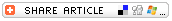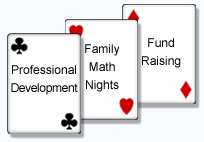## Search formMath Games With Jane and Joanne

# Slide Over## SKILLS

• subtracting 3-digit numbers

• 2

## EQUIPMENT

• playing cards Ace (=1) through 9
• paper and pencil

## GETTING STARTED

Each player starts the game with 1,000 points on their paper. The goal of this game is to get as close to zero as possible.

Player 1 turns over three cards from the deck. She creates a 3-digit number by using the first card drawn to represent the hundreds place, the second card to represent the tens place, and the third card to represent the ones place.

Next, Player 2 turns over three cards. He does the same thing.

Both players subtract the number they created from 1,000 to find the difference.

Players continue to take turns. On subsequent turns, players subtract the new number created by drawn cards from the remaining difference in their calculations. Players must determine if the number can be subtracted in the hundreds place. If the number being subtracted is larger than the number on top, then the player "slides over" the cards. The number that was in the hundreds place is now in the tens place, the number that was in the tens place is now in the ones place, and the number that was in the ones place "slides" out of the game. Now the student can subtract a 2-digit number from the number above it.

When players get to the point that their difference is a 2-digit number, they only need to draw two cards. If the cards they draw are larger than the difference, then they slide over the cards so that the digit in the tens column becomes the digit in the ones column. They subtract that 1-digit number from the previous difference.

As players get close to zero, they can freeze at any time or take a chance by turning over a single card. But if the player turns over a card greater than the difference that remains, the player is out and their opponent wins.

Example
• Player 1 draws the cards 5, 5, and 5. She subtracts the number 555 from 1,000 for a difference of 445.
• Next turn, Player 1 draws the cards 6, 7, and 2. Since 672 is larger than the difference that remains (445), the player "slides over" the cards. She subtracts 67 from 445 for a difference of 378.
• On her third draw, Player 1 draws the cards 1, 7, and a King (=0). She subtracts 170 from 378 for a difference of 208.
• On the fourth turn, Player 1 draws the cards 1, 9, and 1. She subtracts 191 from 208 for a difference of 17.
• On the fifth turn, Player 1 only needs to draw two cards. She draws a 7 and a 5. Since 75 is too large a number to subtract from 17, she "slides over" the 7 into the ones place and subtracts 7 from 17 for a difference of 10.
• On the sixth draw, Player 1 gets a 5. She subtracts 5 from the last remaining difference (10) for a difference of 5.
• Player 1 chooses to freeze at this point.

When both players have frozen, the one closest to zero is the winner.Joanne Currah and Jane Felling have created two best-selling math game books -- Shuffling Into Math (for teachers of grades K-3) and All Hands on Deck (grades 1-9). Each book includes simple instructions for more than 100 math games that help children discover math concepts and reinforce math skills. Education World is pleased to present a selection of those activities in this series.Click to learn more about these books and other exciting products and service from Joanne and Jane's Box Cars and One-Eyed Jacks.

### More About Box Cars and One-Eyed JacksJoanne Currah and Jane Felling created their company, BOX CARS & ONE-EYED JACKS, for the sole purpose of making math fun -- not threatening or frustrating -- for children. Joanne and Jane spend many days each year on the road as they train teachers to make math fun for kids and run Family Math Nights at schools. To learn more about the authors, their books, and the services they provide, visit them at www.boxcarsandoneeyedjacks.com or email them at [email protected].

Education World®